# Test DHT22 Sensors with Arduino and MATLAB

This project shows how to use MATLAB and Arduino to acquire and fix data from a bundle of DHT22 sensors, live plot, and export the results.

IntermediateFull instructions provided7,970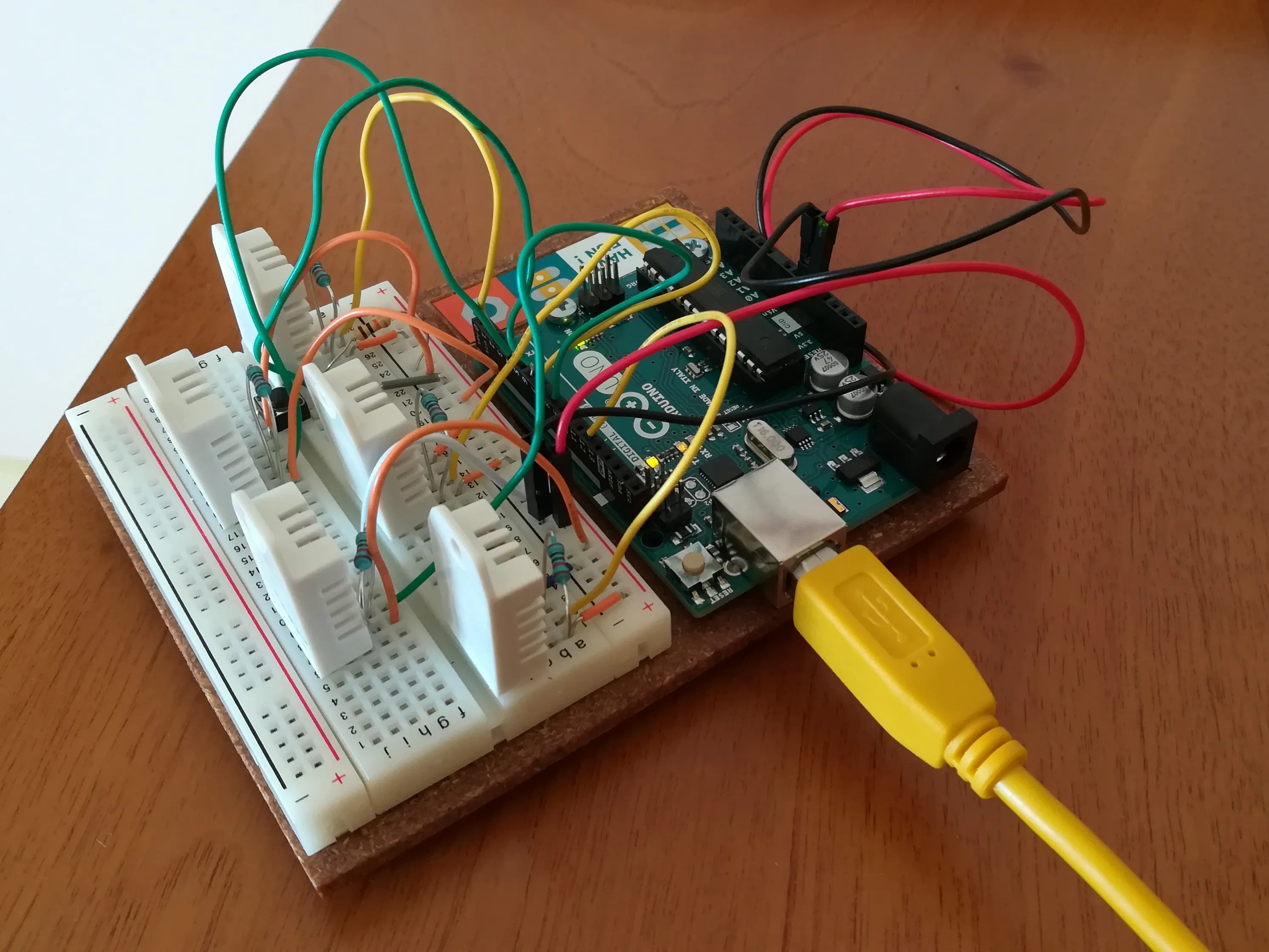## Things used in this project

### Hardware componentsArduino UNO & Genuino UNO but also a Nano will work
×1×1Jumper wires (generic)
×1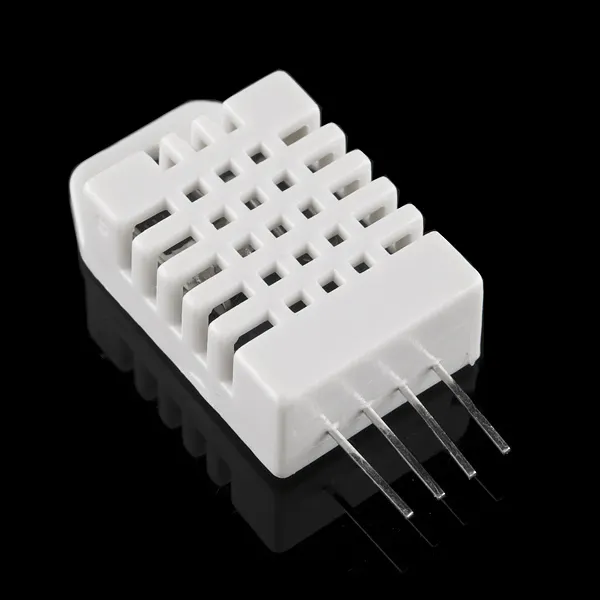DHT22 Temperature Sensor
×5SparkFun Pushbutton switch 12mm not mandatory, you may rely only on time limit
×1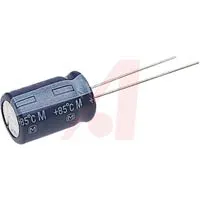Capacitor 10 µF useful to avoid auto reset on serial connection
×1Resistor 1k ohm switch pull-down resistor
×1Resistor 10k ohm usually included in the DHT22 sensor, but nothing wrong happens by adding them
×5

### Software apps and online servicesArduino IDE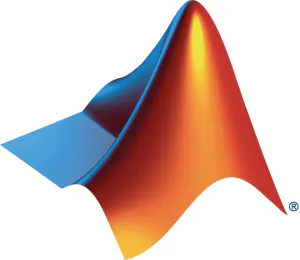MATLAB

## Schematics

### Connections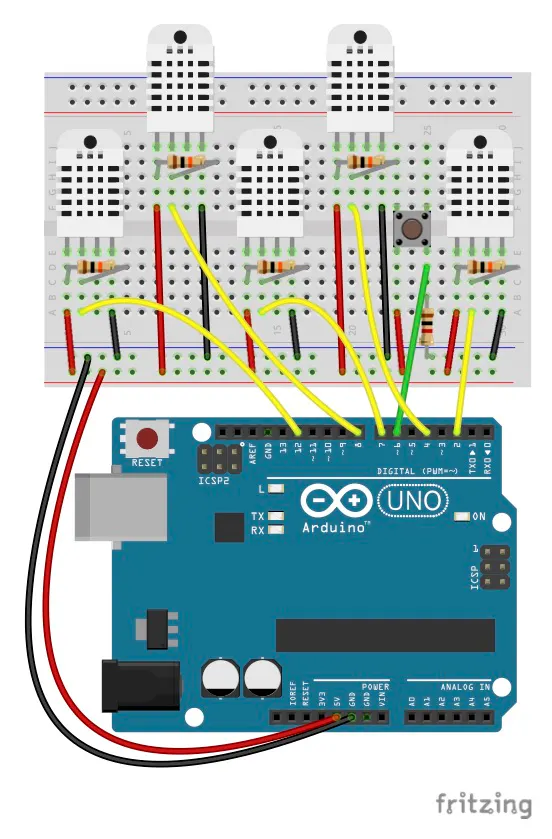## Code

### DHT22_5sensor.ino

Arduino
Arduino sketch to read sensors data and pass them to the computer by the serial port.
```// Libraries
#include <DHT.h>

// Initialize DHT sensor for normal 16mhz Arduino
DHT dht1(2, DHT22);
DHT dht2(4, DHT22);
DHT dht3(7, DHT22);
DHT dht4(8, DHT22);
DHT dht5(12, DHT22);

int const stopPin = 6;
int stopFlag = 0;

// Variables
float hum;  //Stores humidity value
float temp; //Stores temperature value

void setup()
{
pinMode(stopPin, INPUT);
Serial.begin(9600);
dht1.begin();
dht2.begin();
dht3.begin();
dht4.begin();
dht5.begin();
}

void loop()
{
delay(2000);

// Read humidity from the 5 sensors and display it on a single line
Serial.print(hum);
Serial.print(":");
Serial.print(hum);
Serial.print(":");
Serial.print(hum);
Serial.print(":");
Serial.print(hum);
Serial.print(":");
Serial.print(hum);
Serial.print(":");

// Read temperature from the 5 sensors and display it on a single line
Serial.print(temp);
Serial.print(":");
Serial.print(temp);
Serial.print(":");
Serial.print(temp);
Serial.print(":");
Serial.print(temp);
Serial.print(":");

// Check stop condition
if (stopFlag == LOW){
Serial.println(temp);
}
else{
Serial.print(temp);
Serial.println(":999");
}

}
```

### DHT22_5sensor.m

MATLAB
MATLAB code to read serial data from the Arduino. Please check your port number on line 18. First acquisition should be without correction. For the first time leave lines 66 to 68 commented. I have used an external tool to verify temperature and humidity readings and it appeared that sensor number 5 read both correctly in several condition. I have written the humCorr.m function to fix the other sensors readings from the value of sensor number 5. If interested in correction, manipulate humCorr.m function and/or lines 66-68 according to your needs.
```% Script to test a bundle of 5 DHT22 sensors with an Arduino (Uno)
% microcontroller. Acquire data from the sensors until a stop button is
% pressed on the board or a time limit is reached. Plot last 10 minutes of
% live data during acquisition, the entire data set after the acquisition,
% and save these data on a spreadsheet.

close all
instrreset
clear
clc

% Acquisition time (min). Insert inf to disable time limit.
waitTime = 10;

%% Acquire and display live data

% Open serial communication
s = serial('/dev/cu.usbmodem411','BAUD',9600);

figure
color = ['b', 'r', 'g', 'm', 'c', 'b', 'r', 'g', 'm', 'c'];
for i = 1:5
h(i) = animatedline('Color',color(i),'LineWidth',2);
end
axh = gca;
axh.YGrid = 'on';
axh.YLim = [30 80];
xlabel('Time')
ylabel('Humidity (%)')
legend('Sensor 1', 'Sensor 2', 'Sensor 3', 'Sensor 4', 'Sensor 5',...
'Location','NorthWest')

figure
for i = 6:10
h(i) = animatedline('Color',color(i),'LineWidth',2);
end
axt = gca;
axt.YGrid = 'on';
axt.YLim = [10 40];
xlabel('Time')
ylabel('Temperature (\circC)')
legend('Sensor 1', 'Sensor 2', 'Sensor 3', 'Sensor 4', 'Sensor 5',...
'Location','NorthWest')

stop = false;
waitTime = duration(0,waitTime,0);
startTime = datetime('now');
t = datetime('now') - startTime;

while ~stop && t < waitTime

% Read data from serial port
fopen(s);
idn = fscanf(s);
fclose(s);

% Separate data
C = strsplit(idn,':');

% Display data in MATLAB command window
serialData = str2double(C);

% Humidity correction factor from measurement of sensor 5
corrData = serialData;
% First acquisition should be without correction. For the first time leave lines 66 to 68 commented. I have used an external tool to verify temperature and humidity readings and it appeared that sensor number 5 read both correctly in several condition. I have written the humCorr.m function to fix the other sensors readings from the value of sensor 5. If interested in correction, manipulate humCorr.m function and/or the following lines according to your needs.
%for i = 1:4
%   corrData(i) = serialData(i) * humCorr(serialData(5),i);
%end

disp(corrData)

% Get current time
t = datetime('now') - startTime;

% Add points to animation (humidity data)
for i = 1:5
end

% Update axes
axh.XLim = datenum([t-seconds(600) t]);
datetick('x','keeplimits')
drawnow

% Add points to animation (temperature data)
for i = 6:10
end

% Update axes
axt.XLim = datenum([t-seconds(600) t]);
datetick('x','keeplimits')
drawnow

% Check stop condition from serial monitor
if str2double(C{end}) == 999
stop = true;
end
end

% Output message
if stop
disp('Data acquisition ended because the STOP button has been pressed')
else
disp('Data acquisition ended because the TIME limit has been reached')
end

%% Plot the recorded data

for i = 1:5
[~,humLogs(i,:)] = getpoints(h(i));
[timeLogs,tempLogs(i,:)] = getpoints(h(i+5));
end
timeSecs = (timeLogs-timeLogs(1))*24*3600;

figure
subplot(1,2,1)
plot(timeSecs,humLogs,'LineWidth',2)
grid on
ax = gca;
ylim([round(ax.YLim(1)-2), round(ax.YLim(2)+2)])
xlabel('Elapsed time (s)')
ylabel('Humidity (%)')

subplot(1,2,2)
timeSecs = (timeLogs-timeLogs(1))*24*3600;
plot(timeSecs,tempLogs,'LineWidth',2)
hold off, grid on
ax = gca;
ylim([round(ax.YLim(1)-2), round(ax.YLim(2)+2)])
xlabel('Elapsed time (s)')
ylabel('Temperature (\circC)')
legend('Sensor 1', 'Sensor 2', 'Sensor 3', 'Sensor 4', 'Sensor 5',...
'Location','Best')

%% Save results to a file

T = table(timeSecs',humLogs',tempLogs','VariableNames',...
{'Time_s','Relative_Humidity','Temperature_C'});
filename = 'Humidity_and_Temperature_Data.xls';

% Delete previous file, if exists, to avoid append of data
if exist(filename,'file')
delete(filename)
end

% Write table to file
writetable(T,filename)
% Print confirmation to command line
fprintf('Results table with %g humidity and temperature measurements saved to file %s\n',...
length(timeSecs),filename)

%% Summary charts with original data, averaged data, and uncertainty

for sensor = 1:5

% Smooth out readings with moving average filter
smoothHum = smooth(humLogs(sensor,:),25);
smoothTemp = smooth(tempLogs(sensor,:),25);

% Typical accuracy of the humidity sensor
humMax = 1.02 * smoothHum;
humMin = 0.98 * smoothHum;

% Worst accuracy of the humidity sensor
humMaxW = 1.05 * smoothHum;
humMinW = 0.95 * smoothHum;

% Accuracy of the temperature sensor
tempMax = smoothTemp + 0.5;
tempMin = smoothTemp - 0.5;

figure
subplot(1,2,1), hold on
plot(timeSecs,humLogs(sensor,:),'b','LineWidth',2)
plot(timeSecs,smoothHum,'r','LineWidth',1)
plot(timeSecs,humMin,'r--','LineWidth',2)
plot(timeSecs,humMax,'r--','LineWidth',2)
plot(timeSecs,humMinW,'m--','LineWidth',1)
plot(timeSecs,humMaxW,'m--','LineWidth',1)
hold off, grid on, ylim([round(min(humMinW))-2, round(max(humMaxW))+2])
xlabel('Elapsed time (s)')
ylabel('Humidity (%)')
title(['Humidity data, average, and uncertainty for sensor ',num2str(sensor)])

subplot(1,2,2), hold on
plot(timeSecs,tempLogs(sensor,:),'b','LineWidth',2)
plot(timeSecs,smoothTemp,'r','LineWidth',1)
plot(timeSecs,tempMin,'r--','LineWidth',2)
plot(timeSecs,tempMax,'r--','LineWidth',2)
hold off, grid on, ylim([round(min(tempMin))-2, round(max(tempMax))+2])
xlabel('Elapsed time (s)')
ylabel('Temperature (\circC)')
title(['Temperature data, average, and uncertainty for sensor ',num2str(sensor)])

end
```

### humCorr.m

MATLAB
MATLAB function used to correct the humidity readings from the value of one sensor. Not called if lines 66-68 of the main script DHT22_5sensor.m are commented. Ignore or edit this function according to your needs.
```function corrFact = humCorr(rh5,sensNum)
% Humidity correction factor from measurement of sensors 1,2,3,4,5
% rh5 is the relative humidity of sensor number 5
% sensNum is the sensor number (1 to 5)
%
% Data to interpolate:
% RH5 30.843        1.008   1.007   1.065   1.011   1.000
% RH5 53.775		1.077	1.150	1.136	1.059   1.000

data = [ 36.992     1.033	1.056	1.114	1.040   1.000;...
62.333		1.060	1.115	1.170	1.066   1.000];

% check on input
if sensNum ~= 1 && sensNum ~= 2 && sensNum ~= 3 && sensNum ~= 4 && sensNum ~= 5
error('sensNum must be 1 or 2 or 3 or 4 or 5')
end

deltaX = data(2,1) - data(1,1);             % scalar
deltaY = data(2,2:end) - data(1,2:end);     % array 1D
m = deltaY/deltaX;                    % slope of the linear interpolant

corrFact = data(1,sensNum+1) + m(sensNum) * (rh5 - data(1,1));
```

## Credits

### dancili

0 projects • 10 followers